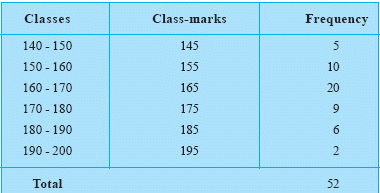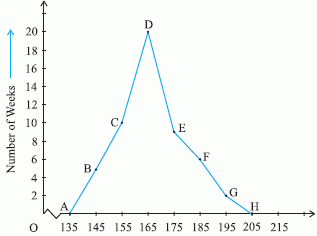Email us to get an instant 20% discount on highly effective K-12 Math & English kwizNET Programs!

#### Online Quiz (WorksheetABCD)

Questions Per Quiz = 2 4 6 8 10

### Middle/High School Algebra, Geometry, and Statistics (AGS)9.18 Frequency Polygon without Histograms

 Frequency Polygons can be drawn independently without drawing the histograms. In drawing a histogram of a given frequency distribution, we take the following steps: Step 1. : If the frequency table is in the inclusive form, we first convert it into an exclusive form and make it a continuous interval. Step 2. :To complete the polygon we assume a class interval with zero frequency preceding the first class interval and a class interval with zero frequency succeeding the last class interval. Step 3. : Taking a suitable scale, we represent class marks along x axis. Step 4. : Taking a suitable scale, we represent frequency along y axis. Step 5. : We plot the corresponding points and join it with the help of line segment. Consider the following example. In a city, the following weekly observations were made in a study on cost of living index :Following is the frequency polygon for the above dataDirections: On the basis of the given information answer the following questions
 Q 1: The lower limit for the fifth class interval is180160190170 Q 2: Write down the corresponding class interval for the class mark 145.170 - 180160 - 170150 - 160140 - 150 Q 3: The maximum frequency corresponds to which class mark?155145140165 Q 4: Frequency Polygons without histograms are drawn with the help of class marks.faseTrue Question 5: This question is available to subscribers only! Question 6: This question is available to subscribers only!